Test: Simpler Notations

# Test: Simpler Notations - Computer Science Engineering (CSE)

Test Description

## 10 Questions MCQ Test Theory of Computation - Test: Simpler Notations

Test: Simpler Notations for Computer Science Engineering (CSE) 2023 is part of Theory of Computation preparation. The Test: Simpler Notations questions and answers have been prepared according to the Computer Science Engineering (CSE) exam syllabus.The Test: Simpler Notations MCQs are made for Computer Science Engineering (CSE) 2023 Exam. Find important definitions, questions, notes, meanings, examples, exercises, MCQs and online tests for Test: Simpler Notations below.
Solutions of Test: Simpler Notations questions in English are available as part of our Theory of Computation for Computer Science Engineering (CSE) & Test: Simpler Notations solutions in Hindi for Theory of Computation course. Download more important topics, notes, lectures and mock test series for Computer Science Engineering (CSE) Exam by signing up for free. Attempt Test: Simpler Notations | 10 questions in 10 minutes | Mock test for Computer Science Engineering (CSE) preparation | Free important questions MCQ to study Theory of Computation for Computer Science Engineering (CSE) Exam | Download free PDF with solutions
 1 Crore+ students have signed up on EduRev. Have you?
Test: Simpler Notations - Question 1

### Given Language: L= {xϵ∑= {a, b} |x has a substring ‘aa’ in the production}. Which of the corresponding representation notate the same?

Detailed Solution for Test: Simpler Notations - Question 1

The states transited has been written corresponding to the transitions as per the row and column. The row represents the transitions made and the ultimate.

Test: Simpler Notations - Question 2

### the row and column. The row represents the transitions made and the ultimate.2.Let u=’1101’, v=’0001’, then uv=11010001 and vu= 00011101.Using the given information what is the identity element for the string?

Detailed Solution for Test: Simpler Notations - Question 2

Identity relation: εw = wε = w, thus the one satisfying the given relation will be the identity element.

Test: Simpler Notations - Question 3

### Which of the following substring will the following notation result?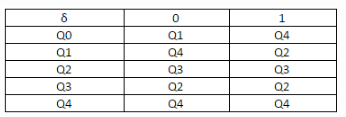Detailed Solution for Test: Simpler Notations - Question 3

The given DFA notation accepts the string of even length and prefix ‘01’.

Test: Simpler Notations - Question 4

Predict the following step in the given bunch of steps which accepts a strings which is of even length and has a prefix=’01’
δ (q0, ε) =q0 < δ(q0,0) =δ (δ (q0, ε),0) =δ(q0,0) =q1 < _______________

Detailed Solution for Test: Simpler Notations - Question 4

Here, δ refers to transition function and results into new state or function when an transition is performed over its state.

Test: Simpler Notations - Question 5

Fill the missing blank in the given Transition Table:
Language L= {xϵ∑= {0,1} |x accepts all the binary strings not divisible by 3}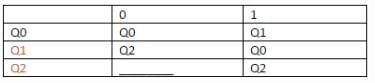Detailed Solution for Test: Simpler Notations - Question 5

The tabular representation of DFA is quite readable and can be used to some ore complex problems. Here, we need to form the transition graph and fill up the given blank..

Test: Simpler Notations - Question 6

.Which among the following is the missing transition in the given DFA?
L= {xϵ∑= {a, b} | x starts with a and ends with b}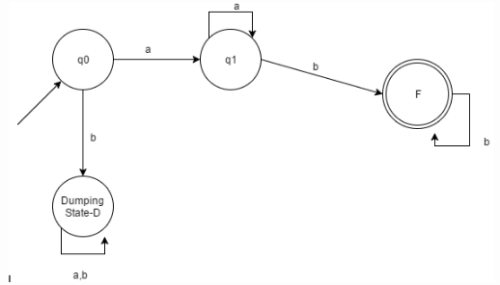Detailed Solution for Test: Simpler Notations - Question 6

For the given Language, the transition missing is δ (F, a) =q1.

Test: Simpler Notations - Question 7

The complement of a language will only be defined when and only when the __________ over the language is defined.

Detailed Solution for Test: Simpler Notations - Question 7

It is not possible to define the complement of a language without defining the input alphabets. Example: A language which does not consist of substring ‘ab’ while the complement would be the language which does contain a substring ‘ab’.

Test: Simpler Notations - Question 8

Which among the following is not notated as infinite language?

Detailed Solution for Test: Simpler Notations - Question 8

Factorial, here is the most appropriate non-infinite domain. Otherwise, palindrome and reverse have infinite domains.

Test: Simpler Notations - Question 9

Which among the following states would be notated as the final state/acceptance state?L= {xϵ∑= {a, b} | length of x is 2}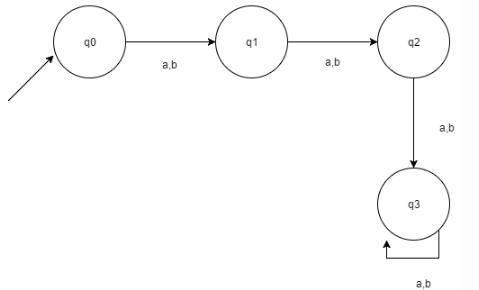Detailed Solution for Test: Simpler Notations - Question 9

According to the given language, q2 Is to become the final/acceptance state in order to satisfy.

Test: Simpler Notations - Question 10

Which of the following are the final states in the given DFA according to the Language given.?
L= {xϵ∑= {a, b} |length of x is at most 2}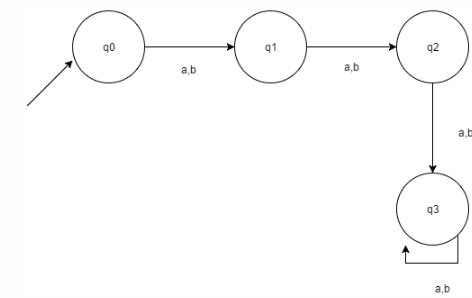Detailed Solution for Test: Simpler Notations - Question 10

According to the given language, the length is at most 2, thus the answer is found accordingly.

## Theory of Computation

18 videos|56 docs|44 tests
Information about Test: Simpler Notations Page
In this test you can find the Exam questions for Test: Simpler Notations solved & explained in the simplest way possible. Besides giving Questions and answers for Test: Simpler Notations, EduRev gives you an ample number of Online tests for practice

## Theory of Computation

18 videos|56 docs|44 tests